Project tutorial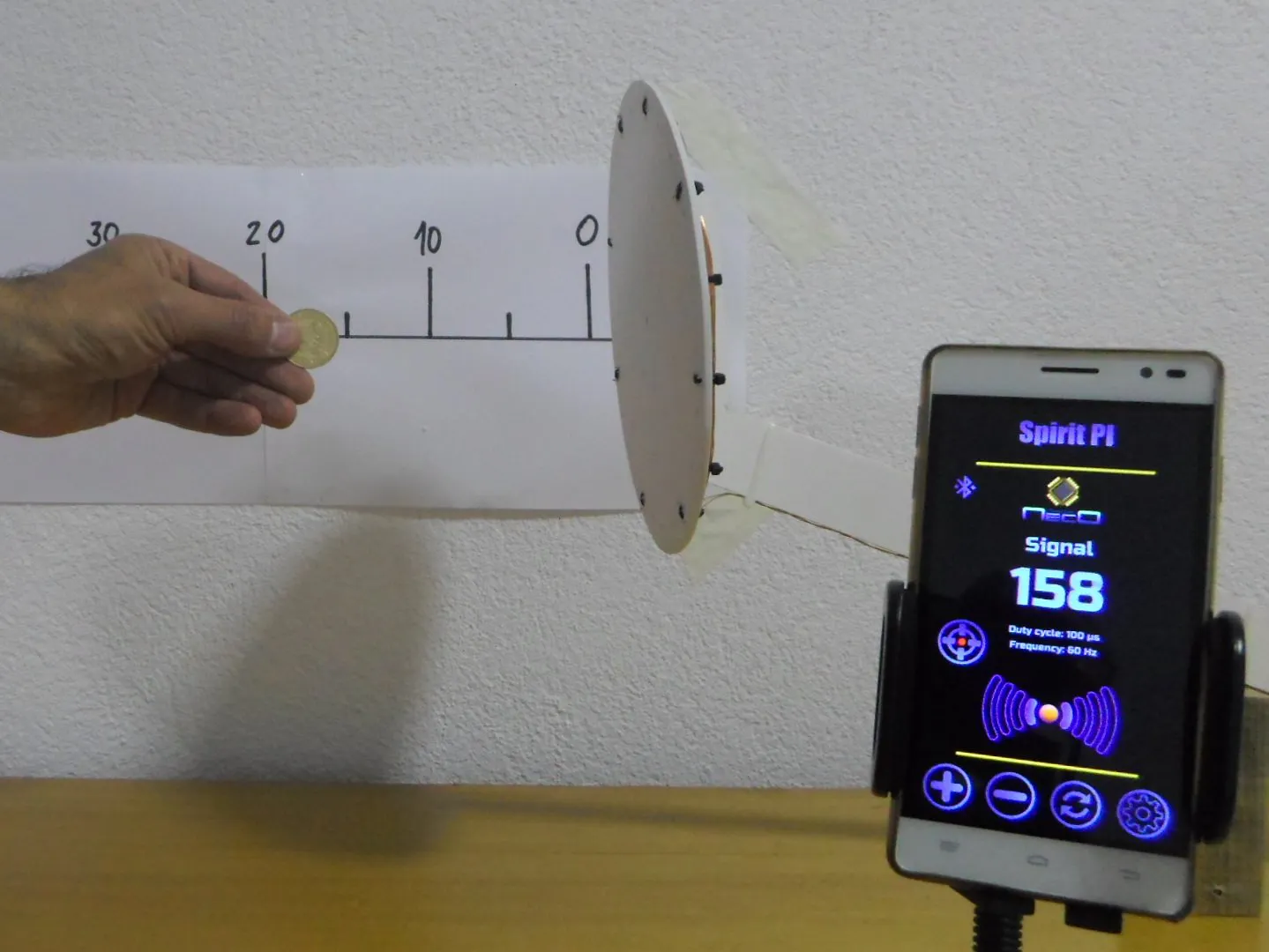# DIY Arduino Based Pulse Induction Metal Detector © GPL3+

The detector can detect a small metal coin at a distance of 15 centimeters.

• 11,704 views
• 19 respects

## Components and suppliesArduino Nano R3
×1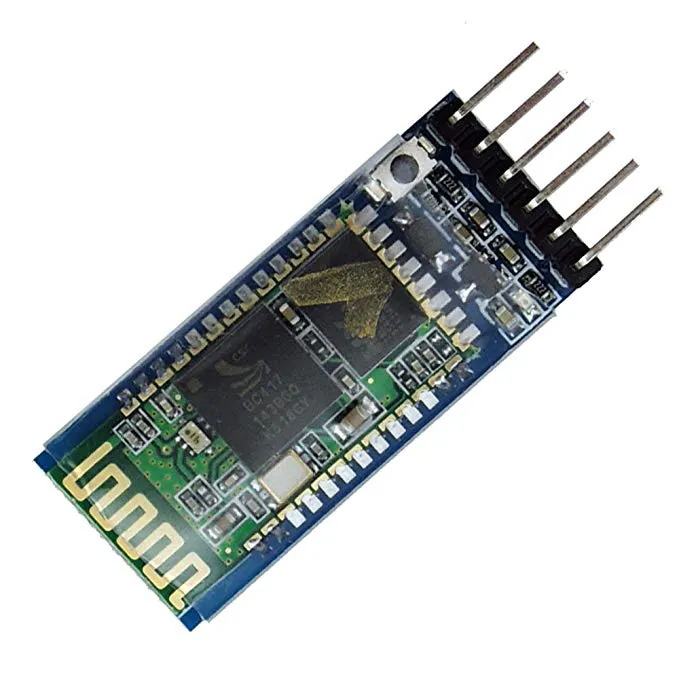HC-05 Bluetooth Module
×1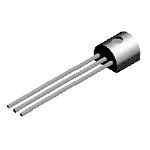General Purpose Transistor PNP
×1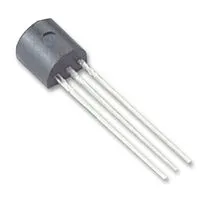General Purpose Transistor NPN
×1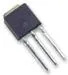Power MOSFET N-Channel
×1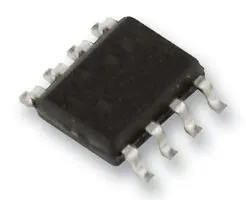Operational Amplifier, Op Amp + Comparator + Reference
×1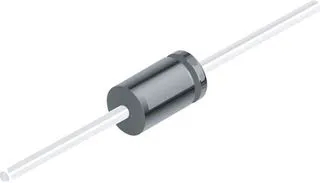1N4148 – General Purpose Fast Switching
×2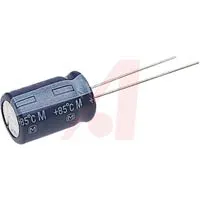Capacitor 10 µF
×1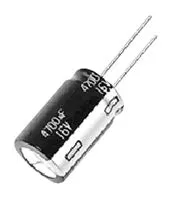Capacitor 1 µF
×1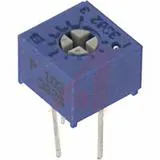Single Turn Potentiometer- 100k ohms
×1
 Single Turn Potentiometer-47k
×1
 12 resistors with different values
×1

## Necessary tools and machinesSoldering iron (generic)

Pulse Induction (PI) systems use a single coil as both transmitter and receiver. This technology sends powerful, short bursts (pulses) of current through a coil of wire. Each pulse generates a brief magnetic field. When the pulse ends, the magnetic field reverses polarity and collapses very suddenly, resulting in a sharp electrical spike. This spike lasts a few microseconds and causes another current to run through the coil. This current is called the reflected pulse and is extremely short, lasting only about 30 microseconds. Another pulse is then sent and the process repeats. If a piece of metal comes inside the range of the magnetic field lines, the receive coil can detect a change in both amplitude and phase of the received signal. The amount of amplitude change and phase change is an indication for the size and distance of the metal, and can also be used to discriminate between ferrous and non-ferrous metals.

I found a good example of a PI detector on the site of the N.E.C.O. projects. This metal detector is a symbiosis of Arduino and Android. On the Play Store you can download the free version of application "Spirit PI," which is fully functional, but you can also buy a pro version that has several great options. The communication between the smartphone and the arduino is done with the bluetooth module HC 05, but you can use any bluetooth adapter on which you have to turn baud rate to 115200. The scheme is given in the figure below. I made several minor modifications to the original scheme in order to improve the features of the device. You can find original schematic at N.E.C.O.-s web:

https://neco-desarrollo.es/pirat-wireless-metal-detector

In the place of a 150 ohm resistor, I put a trimer potentiometer with a value of 47 Kohms. This trimer regulates the current through the coil. By increasing its value, the current through the coil increases and the sensitivity of the device increases.Second modification is trimmer pot 100kOhm instead resistor 62k in original. With this trimer, we set the voltage of about 4.5V to A0 input on Arduino, because I noticed that for a different operational amplifiers and operating voltages, the value of this resistor should be different.

In this particular case, for powering device I use a 4 lithium ion battery connected in a series so the voltage is something greater than 15v. Because Arduino accepts a maximum of 12V input voltage, I put a stabilizer for 5V (7805) mounted on the small heatsink for powering the Arduino directly to +5v pin.

The coil is made from isolated copper wire with a diameter of 0.4 mm and contains 25 windings in the shape of a circle with a diameter of 19 centimeters.In the final workmanship, it is necessary to ensure that there are no metal objects close to the coil (the elements are to be glued with glue, and that no screws)

As you can see on the video, a small metal coin can be detected at a distance of 10-15 centimeters, while a larger metal object of 30-40 centimeters and more. These are excellent results, taking into account that the making and setting of the device are relatively simple.

## Code

##### codeArduino
```#include <EEPROM.h>
//#include <SoftwareSerial.h>
//int bluetoothTx = 5;
//int bluetoothRx = 6;//blutoth module terminal RX
//SoftwareSerial bluetooth(bluetoothTx, bluetoothRx);
#define pulsePine 2
int timer = 200;
const int button1 = 12;
int buttonState1 = 0;

char data;
//EEPROM variables
int stored_value;
int duty_cycle;
int duty_cycle_temp;
int freq;
int freq_temp;
int duty_def_value = 10;
int freq_def_value = 60;
//Balance variables
int value_count = 0;
int value_count_def = 100;
int balance_value = 0;
int balance_value_temp = 0;
//****
unsigned long startMillis;
unsigned long currentMillis;
long period = 100000;  //the value is a number of microseconds
//Measuring of level of the battery
float resistencia1 = 101000; //Resistencia de 100K para medir la tencion (Voltios)/Resistance of 100k for test volts
float resistencia2 = 10000; //Resistencia de 10k para medir la tencion (Voltios)/Resistance 10k for test volts
float const arefVolt=4.8f; //pin "4.9v" SET EXACT VALUE HERE
float voutv;
float vinv;
unsigned long startMillisVolts;
unsigned long currentMillisVolts;
long periodVolts = 2000;  //the value is a number of microseconds
int sensorValue ;

void setup() {
//analogReference(INTERNAL);
Serial.begin(115200);
duty_cycle = stored_value;
freq = stored_value;
if(duty_cycle == 0 || duty_cycle == 255){
duty_cycle = stored_value;
}

if(freq == 0 || freq == 255 ){
freq = stored_value;
}

pinMode(pulsePine, OUTPUT);

}

void loop() {
currentMillis = micros();
currentMillisVolts = millis();

if(Serial.available()>0)
{
setDutyAndFreq  (data);

}

if (currentMillis - startMillis >= period)
{

period = 1000000 / freq;
// Serial.println(period);
digitalWrite(pulsePine, HIGH);
duty_cycle_temp = duty_cycle * 10;
delayMicroseconds(duty_cycle_temp);
digitalWrite(pulsePine, LOW);

for(int i = 0;i < 3; i++){

}

delayMicroseconds(10);
sensorValue = sensorValue / 5;
sendData();

startMillis = currentMillis;
}
// Lectura voltios
if (currentMillisVolts - startMillisVolts >= periodVolts)
{
lecturaVoltios();
//Serial.println("Lectura voltios");
startMillisVolts = currentMillisVolts;
}

}

{

}
{

return stored_value;

}
void setDutyAndFreq  (char valor)
{
//"n" valor para aumentar duty cycle
//"m" valor para disminuir duty cycle
//"j" valor para aumentar la frequencia
//"k" valor para des,inuir la frequencia
//"+" valor para aumentar el balance
//"-" valor para desminuir el balance
if(valor == 'n')
{
// Serial.println("n Recived");
duty_cycle = stored_value;
duty_cycle = duty_cycle + 1;

}
else if(valor == 'm')
{
//  Serial.println("m Recived");
duty_cycle = stored_value;
duty_cycle = duty_cycle - 1;

}
else if(valor == 'j')
{
//    Serial.println("j Recived");
freq = stored_value;
freq = freq + 10;

}
else if(valor == 'k')
{
//   Serial.println("k Recived");
freq = stored_value;
freq = freq - 10;

}
else if(valor == 'p')
{
//  Serial.println("m Recived");

}

}
//Volt function
void lecturaVoltios(){
vinv=0.0f;
voutv=0.0f;
for (int i=0;i < 100;i++){

voutv = (analogRead(A7) * arefVolt) / 1023;  //Lee el voltaje de entrada
vinv += voutv / (resistencia2 / (resistencia1 + resistencia2));  //Fórmula del divisor resistivo para el voltaje final
if(vinv < 0.9){
vinv=0.0f;
}

}
vinv = vinv/100;

}
void sendData()

{
/* Serial.print("<");
Serial.print(sensorValue);
Serial.print("/");
Serial.print(freq);
Serial.print("/");
Serial.print( duty_cycle);
Serial.print("/");
Serial.print( vinv);
Serial.print(">");
Serial.println();*/
String data = "<";
data +=sensorValue;
data +="/";
data +=freq;
data +="/";
data +=duty_cycle;
data +="/";
data +=vinv;
data +=">";
/* bluetooth.print("<");
bluetooth.print(sensorValue);
bluetooth.print("/");
bluetooth.print(freq);
bluetooth.print("/");
bluetooth.print( duty_cycle);
bluetooth.print("/");
bluetooth.print( vinv);
bluetooth.print(">");*/
Serial.println(data);
}
```

## Schematics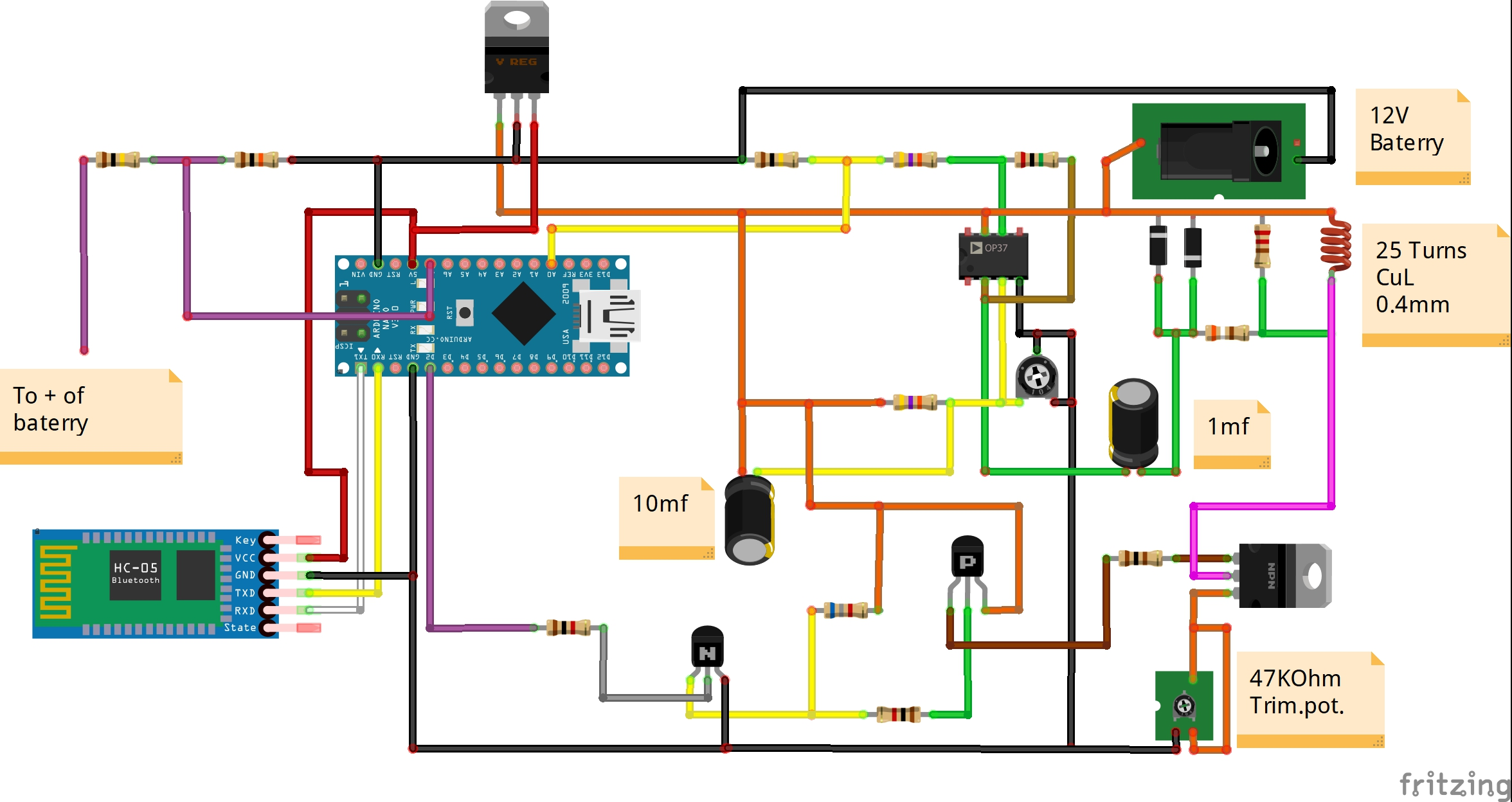#### Author##### Mirko Pavleski
• 44 projects
• 145 followers

April 19, 2019

#### Members who respect this project

See similar projects
you might like

#### FoxyPI - Arduino Nano-Based Pulse Metal Detector

Project showcase by FoxyLab

• 13,762 views
• 21 respects

#### DIY 24x6 (144 Big LEDs) Matrix with Scrolling Text

Project tutorial by Mirko Pavleski

• 2,480 views
• 1 comment
• 11 respects

#### DIY Sensitive ADXL335 Earthquake Detector

Project tutorial by Mirko Pavleski

• 2,055 views
• 1 comment
• 8 respects

#### MobBob: DIY Arduino Robot Controlled by Android Smartphone

Project tutorial by Mirko Pavleski

• 4,580 views
• 39 respects

#### Metal Detector Using Frequency Counter and OLED Display

Project tutorial by Andrius Purr

• 3,009 views
• 1 comment
• 19 respects

#### Arduino Bluetooth-Controlled Matrix with Scrolling Text

Project tutorial by Mirko Pavleski

• 10,040 views